# How to Create Correlation Heatmap in R

• Last Updated : 24 Feb, 2022

In this article let’s check out how to plot a Correlation Heatmap in R Programming Language.

Analyzing data usually involves a detailed analysis of each feature and how it’s correlated with each other. It’s essential to find the strength of the relationship between each feature or in other words how two variables move in association to each other. If the variables grow together in the same direction it’s a positive correlation otherwise a negative correlation. This correlation can be visualized via various graphs such as scatter plots etc.

Let’s load the environmental dataset and view the first 6 rows of the data using the head( ) function.

## R

 `# Loading package,data and``# viwing 1st 6 rows of data` `install.packages``(``"lattice"``)``library``(lattice)` `# Load the New York City``# environmental dataset.``data``(environmental)` `data <-environmental``head``(data)`

Output: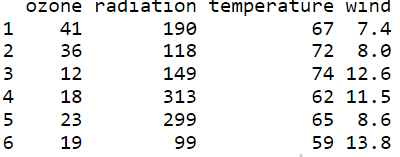## Create Correlation Matrix

Let’s create a correlation matrix for our data using cor( ) function and round each value to 2 decimal places. This matrix can be used to easily create the heatmap.

## R

 `# create a coorelation matrix of the data` `# rounding to 2 decimal places``corr_mat <- ``round``(``cor``(data),2) ``head``(corr_mat)`

Output: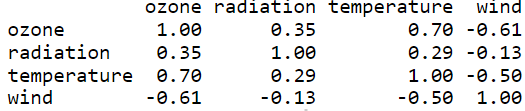## Correlation Heatmap using ggplot2

Using ggplot2 let’s visualize correlation matrix on a heatmap.

Function: ggplot(data = NULL, mapping = aes(), … , environment = parent.frame())

Arguments:

• data – Default dataset to use for plot.
• mapping – Default list of aesthetic mappings to use for plot
• environment – DEPRECATED. Used prior to tidy evaluation

Let’s reduce the size of the correlation matrix by plotting the heatmap using melt( ) function and using ggplot to plot the heatmap. From this heatmap we can easily interpret which variables/features are more correlated and use them for in-depth data analysis. The ggplot function takes in a reduced correlation matrix and aesthetic mappings.

## R

 `# Install and load reshape2 package``install.packages``(``"reshape2"``)``library``(reshape2)` `# creating correlation matrix``corr_mat <- ``round``(``cor``(data),2)` `# reduce the size of correlation matrix``melted_corr_mat <- ``melt``(corr_mat)``# head(melted_corr_mat)` `# plotting the correlation heatmap``library``(ggplot2)``ggplot``(data = melted_corr_mat, ``aes``(x=Var1, y=Var2,``                                   ``fill=value)) +``geom_tile``()`

Output: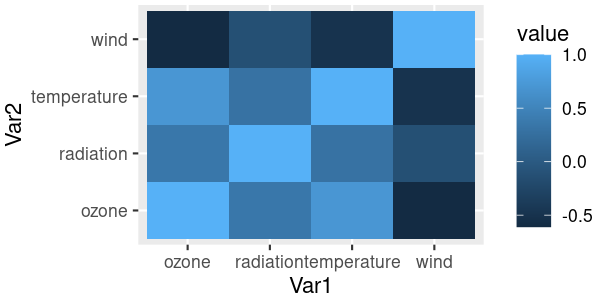## Reorder the Correlation matrix and Plot Heatmap

Reordering or sorting the correlation matrix with respect to coefficient helps us to easily identify patterns between the features/variables. Let’s check out how to reorder the correlation matrix using hclust( ) function by clustering the features hierarchically(hierarchical clustering).

## R

 `# Code to plot a reorederd heatmap` `# Install and load reshape2 package``install.packages``(``"reshape2"``)``library``(reshape2)` `# creating correlation matrix``corr_mat <- ``round``(``cor``(data),2)` `# reorder corr matrix``# using corr coefficient as distance metric``dist <- ``as.dist``((1-corr_mat)/2)` `# hierarchical clustering the dist matrix``hc <- ``hclust``(dist)``corr_mat <-corr_mat[hc\$order, hc\$order]` `# reduce the size of correlation matrix``melted_corr_mat <- ``melt``(corr_mat)``#head(melted_corr_mat)` `#plotting the correlation heatmap``library``(ggplot2)``ggplot``(data = melted_corr_mat, ``aes``(x=Var1, y=Var2, fill=value)) +``geom_tile``()`

Output: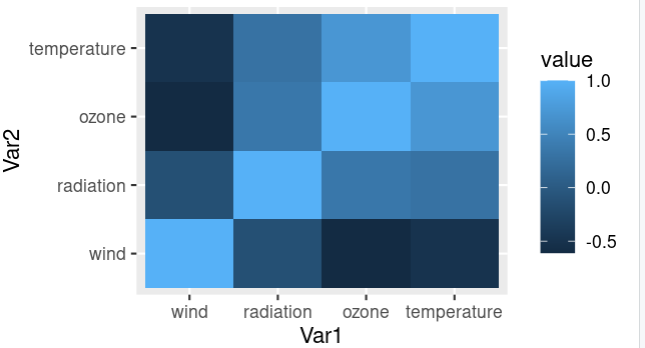## Adding Correlation coefficients to Heatmap

Correlation coefficients are a measure that represents how strong the relationship is between two variables. The higher the absolute value of the coefficient, the higher is the correlation.

Let’s visualize a correlation heatmap along with correlation coefficients on the map using the “value” column in the correlation matrix as text. Using geom_text( ) function annotations can be added on the heatmap and use “value” as labels.

## R

 `# Install and load reshape2 package``install.packages``(``"reshape2"``)``library``(reshape2)` `# creating correlation matrix``corr_mat <- ``round``(``cor``(data),2)` `# reduce the size of correlation matrix``melted_corr_mat <- ``melt``(corr_mat)``head``(melted_corr_mat)` `# plotting the correlation heatmap``library``(ggplot2)``ggplot``(data = melted_corr_mat, ``aes``(x=Var1, y=Var2,``                                   ``fill=value)) +``geom_tile``() +``geom_text``(``aes``(Var2, Var1, label = value),``          ``color = ``"black"``, size = 4)`

Output: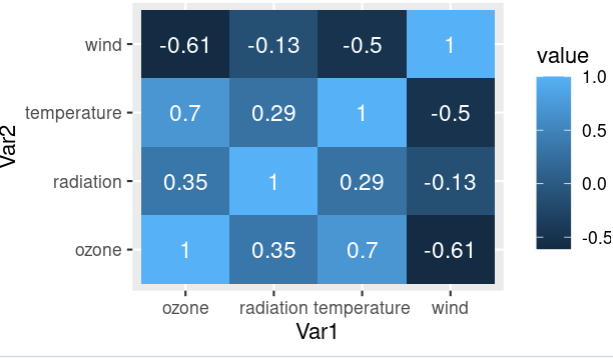## Correlation Heatmap using heatmaply

Let’s use the heatmaply package in R to plot a correlation heatmap using the heatmaply_cor( ) function. Correlation of the data is the input matrix with “Features” column as x and y axis parameters.

Function: heatmaply_cor(x, limits = c(-1, 1), xlab, ylab, colors = cool_warm,k_row, k_col …)

Arguments:

• x – can either be a heatmapr object, or a numeric matrix
• limits – a two dimensional numeric vector specifying the data range for the scale
• colors – a vector of colors to use for heatmap color
• k_row – an integer scalar with the desired number of groups by which to color the dendrogram’s
• branches in the rows
• k_col – an integer scalar with the desired number of groups by which to color the dendrogram’s branches in the columns
• xlab – A character title for the x axis.
• ylab – A character title for the y axis.

## R

 `# Load and install heatmaply package``install.packages``(``"heatmaply"``)``library``(heatmaply)` `# plotting corr heatmap``heatmaply_cor``(x = ``cor``(data), xlab = ``"Features"``,``              ``ylab = ``"Features"``, k_col = 2, k_row = 2)`

Output: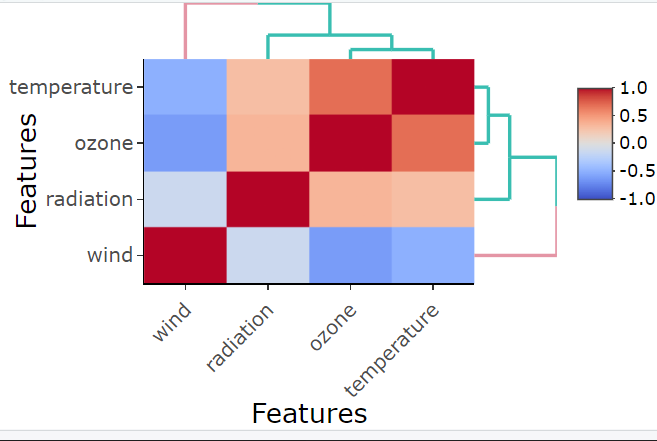## Correlation Heatmap using ggcorplot

Let’s use the ggcorplot package in R to plot a correlation heatmap using ggcorrplot( ) function. The correlation matrix of the data is given as the input corr matrix.

Function: ggcorrplot(corr,method = c(“square”, “circle”) … )

Arguments:

• corr – the correlation matrix to visualize
• method – character, the visualization method of correlation matrix to be used

## R

 `# load and install ggcorplot``install.packages``(``"ggcorplot"``)``library``(ggcorrplot)` `# plotting corr heatmap``ggcorrplot::``ggcorrplot``(``cor``(data))`

Output: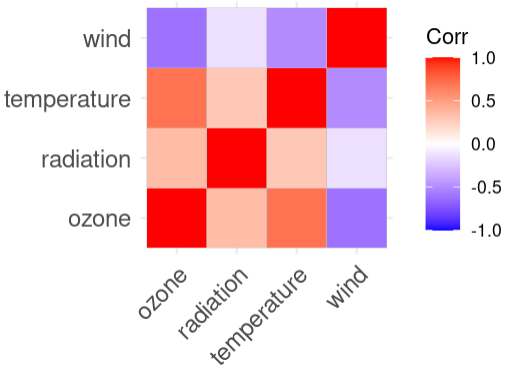## Plotting the lower triangle of the correlation heatmap

Let’s check out how to plot the lower triangle of the correlation heatmap and visualize it. This can be done by replacing the upper triangle values of the correlation matrix as NA and then this matrix is reduced by melting process and plotted.

## R

 `# get the corr matrix``corr_mat <- ``round``(``cor``(data),2)` `# replace NA with upper triangle matrix``corr_mat[``upper.tri``(corr_mat)] <- ``NA` `# reduce the corr matrix``melted_corr_mat <- ``melt``(corr_mat)` `# plotting the corr heatmap``library``(ggplot2)``ggplot``(data = melted_corr_mat, ``aes``(x=Var1,``                                   ``y=Var2,``                                   ``fill=value)) +``  ``geom_tile``()`

Output: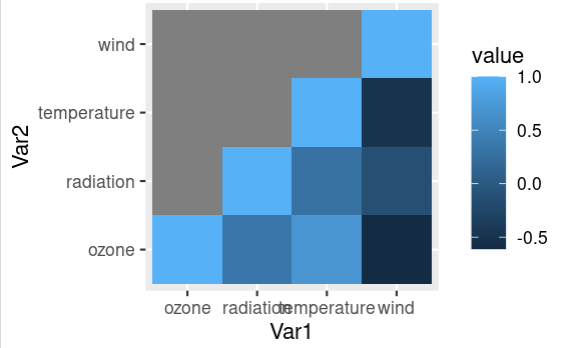## Plotting the upper triangle of the correlation heatmap

Let’s check out how to plot the upper triangle of the correlation heatmap and visualize it. This can be done by replacing the lower triangle values of the correlation matrix as NA and then this matrix is reduced by melting process and plotted.

## R

 `# get the corr matrix``corr_mat <- ``round``(``cor``(data),2)` `# replace NA with lower triangle matrix``corr_mat[``lower.tri``(corr_mat)] <- ``NA` `# reduce the corr matrix``melted_corr_mat <- ``melt``(corr_mat)` `# plotting the corr heatmap``library``(ggplot2)``ggplot``(data = melted_corr_mat, ``aes``(x=Var1, y=Var2,``                                   ``fill=value)) +``  ``geom_tile``()`

Output: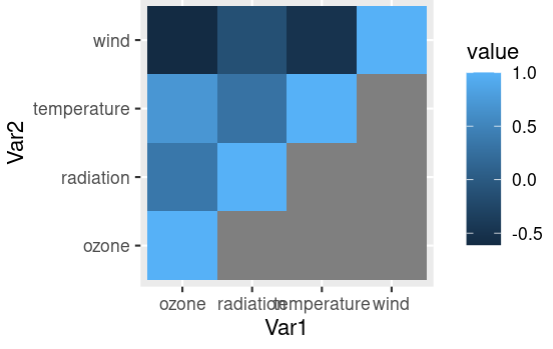## Creating an Interactive Correlation Heatmap

An interactive plot shows detailed information of each data point when the user hovers on the plot. Let’s check out how to plot an interactive correlation heatmap using the correlation matrix and p-value matrix. ggplotly( ) function takes in a correlation matrix of the data and gives an interactive heatmap plot and the details can be viewed on hovering on the map.

Function: ggplotly( p = ggplot2::last_plot(),   width = NULL,  height = NULL … )

Arguments:

• p – a ggplot object
• width – Width of the plot in pixels (optional, defaults to automatic sizing)
• height – Height of the plot in pixels (optional, defaults to automatic sizing)

## R

 `# install and load the plotly package``install.packages``(``"plotly"``)``library``(plotly)``library``(ggcorrplot)` `# create corr matrix and``# coressponding p-value matrix``corr_mat <- ``round``(``cor``(data),2)``p_mat <- ``cor_pmat``(data)` `# plotting the interactive corr heatmap``corr_mat <- ``ggcorrplot``(``  ``corr_mat, hc.order = ``TRUE``, type = ``"lower"``,``  ``outline.col = ``"white"``,``  ``p.mat = p_mat``)` `ggplotly``(corr_mat)`

Output: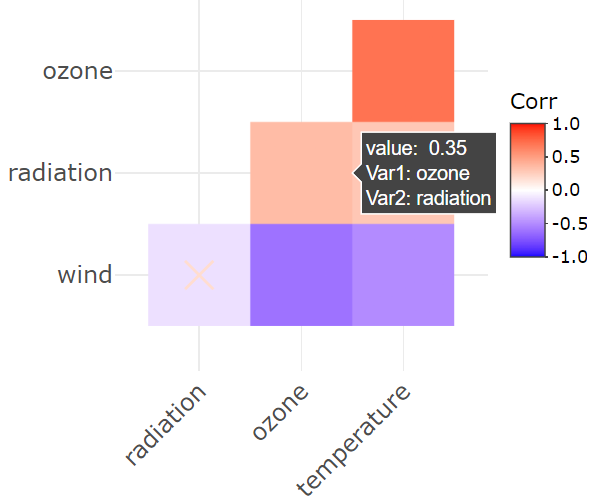My Personal Notes arrow_drop_up